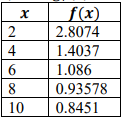# MA.912.AR.5.8Export Print
Given a table, equation or written description of a logarithmic function, graph that function and determine its key features.

### Clarifications

Clarification 1: Key features are limited to domain; range; intercepts; intervals where the function is increasing, decreasing, positive or negative; end behavior; and asymptotes.

Clarification 2: Instruction includes representing the domain and range with inequality notation, interval notation or set-builder notation.

General Information
Subject Area: Mathematics (B.E.S.T.)
Strand: Algebraic Reasoning
Status: State Board Approved

## Benchmark Instructional Guide

### Terms from the K-12 Glossary

• Coordinate plane
• Domain
• Exponential function
• Function
• Function notation
• Logarithmic function
• Range
• $x$-intercept
• $y$-intercept

### Vertical Alignment

Previous Benchmarks

Next Benchmarks

### Purpose and Instructional Strategies

In Algebra I, students graph exponential functions and determine their key features, including asymptotes and end behavior. In Math for College Algebra, students develop an understanding of the inverse relationship of exponential and logarithmic functions.
• Instruction includes making the connection between exponents and logarithms to compare the two in terms of functions (MTR.5.1)
• Instruction includes the use of $x$-$y$ notation and function notation.
• Instruction in Algebra I included representing domain, range and constraints using words, inequality notation and set-builder notation. In Math for College Algebra, instruction also includes interval notation.
• Words
• If the domain is all real numbers, it can be written as “all real numbers” or “any value of $x$, such that $x$ is a real number.”
• Inequality notation
• If the domain is all values of $x$ greater than 2, it can be represented as $x$ >2.
• Set-builder notation
• If the range is all values of $y$ less than or equal to zero, it can be represented as {$y$|$y$ ≤ 0} and is read as “all values of $y$ such that $y$ is less than or equal to zero.”
• Interval notation
• If the domain is all values of $x$ less than or equal to 3, it can be represented as (−∞, 3]. If the domain is all values of $x$ greater than 3, it can be represented as (3, ∞). If the range is all values greater than or equal to −1 but less than 5, it can be represented as [−1, 5).
• Instruction includes the use of appropriately scaled coordinate planes, including the use of breaks in the $x$- or $y$-axis when necessary.
• Instruction includes functions with various bases, including base 10 and base $e$.

### Common Misconceptions or Errors

• Students may not fully understand how to interpret proper function notation when determining the key features of a logarithmic function.
• Students may not fully understand how to use interval notation.
• When describing intervals where functions are increasing, decreasing, positive or negative, students may represent their interval using the incorrect variable. In these cases, ask reflective questions to help students examine the meaning of the domain and range in the problem.
• Students may miss the need for compound inequalities in their intervals. In these cases, refer to the graph of the function to help them discover areas in their interval that would not make sense in context.

• Given $f$($x$) =log3($x$).
• Part A. Graph the function.
• Part B. State the domain of the function.
• Part C. State the range of the function.
• Part D. Determine the asymptote(s).

### Instructional Items

Instructional Item 1
• Below is a table with values for $f$($x$) = log7($x$• Part A. Graph the function.
• Part B. State the domain of the function.
• Part C. State the range of the function.
• Part D. Determine the asymptote(s).

*The strategies, tasks and items included in the B1G-M are examples and should not be considered comprehensive.

## Related Courses

This benchmark is part of these courses.
1200330: Algebra 2 (Specifically in versions: 2014 - 2015, 2015 - 2022, 2022 and beyond (current))
1200340: Algebra 2 Honors (Specifically in versions: 2014 - 2015, 2015 - 2022, 2022 and beyond (current))
1200400: Foundational Skills in Mathematics 9-12 (Specifically in versions: 2014 - 2015, 2015 - 2022, 2022 and beyond (current))
7912095: Access Algebra 2 (Specifically in versions: 2016 - 2018, 2018 - 2019, 2019 - 2022, 2022 and beyond (current))
1200710: Mathematics for College Algebra (Specifically in versions: 2022 and beyond (current))

## Related Access Points

Alternate version of this benchmark for students with significant cognitive disabilities.
MA.912.AR.5.AP.8: Given an equation of a logarithmic function, select the graph of that function.

## Related Resources

Vetted resources educators can use to teach the concepts and skills in this benchmark.

## Student Resources

Vetted resources students can use to learn the concepts and skills in this benchmark.

## Parent Resources

Vetted resources caregivers can use to help students learn the concepts and skills in this benchmark.